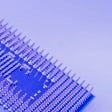# How to Increase Computational Efficiency for PReLU in CUDA — OneFlow Performance Optimization

Written by Zekang Zheng; Translated by Yanjun Hu, Jiali Shen

PReLU is an activation function that is frequently used in InsightFace. It has two operating modes: PReLU(1) and PReLU(channels). For the latter, PReLU is equivalent to a binary broadcast operation. In this article, we are going to talk about optimizing the broadcast operations in CUDA.

PReLU is an activation function that is frequently used in InsightFace. It has two operating modes:

1. PReLU(1). The shape of the weight alpha is (1, ). In this case, PReLU is equivalent to an elementwise operation.
2. PReLU(channels). The shape of the weight alpha is (channels, ), and its size is the same as that of the “C” in the (N, C, H, W) of input tensor. In this case, PReLU is equivalent to a binary broadcast operation.

InsightFace adopts the second mode of PReLU. We wrote about how to optimize CUDA elementwise operationsbefore. Today, we are going to talk about optimizing the broadcast operations in CUDA.

# 1 Naïve implementation

Here is a naïve implementation. Firstly, get the index of the current element-“x” in the loop. Secondly, infer the index of the corresponding alpha weight. Thirdly, return results by seeing if “x”>0: if “x”>0, return “x”; if “x”<0, return “alpha*x”. The code is as follows:

Note:

• `inner_size` refers to the product of the values of the dimensions following the channel dimension. Take the `NCHW` format as an example: `inner_size=H*W`.
• `alpha_size` refers to the size of the channel dimension.

In CUDA, integer division comes with high computational costs. As you can find in the CUDA Toolkit Documentation (Chapter 5.4.1 Arithmetic Instructions):

Integer division and modulo operation are costly as they compile to up to 20 instructions.

Calculating the index of alpha involves one integer division and one modulo operation. These computations represent half of the workload of the kernel. Thus, in what follows, we are going to tell you how to reduce integer divisions and modulo operations while increasing read/write bandwidth using a vectorized method.

# 2 Optimizing by PackType vectorization

Here is a simple case: if the tensor shape being input is (1,2,4,4), then its operating mode will be PReLU(2).

What’s obvious is that the input on H and W dimensions is continuous. In this situation, if `inner_size` is divisible by pack size, the elements in a pack will be applied to the same alpha weight, just as the following figure shows:

In this way, vectorization operations are advisable to improve the read/write bandwidth utilization. And the elements in each pack only need to be calculated once, which cuts down considerable computations, since we no longer need to calculate the elements one by one. The code is as follows:

Let’s compare the results before and after an optimization operation offered by Nsight Compute. After testing data (96, 64, 112, 112) on the A100–40GB GPU, we got the performance results of two kernels as shown in the following figure: the blue one is optimized by vectorization, while the green one is naively implemented. It’s clear that an optimization operation efficiently reduces 20%-30% of calculations and boosts throughput by 30%. Besides, the optimized kernel’s bandwidth is up to 1350GB/s, which gets very close to A100’s theoretical limit.

However, not all tensor shapes support a vectorization operation. If the `inner_size` cannot be divisible by its corresponding `pack_size`, the shape can only settle for a naive implementation.

# 3 Benchmark

When conducting a benchmark test on the NVIDIA A100–40GB GPU, we compared the performance of OneFlow and PyTorch when they deal with different tensor shapes in the InsightFace library. And the testing result is as follows:

We can see that OneFlow, empowered by the optimized activation function-PReLU, delivers better performance than PyTorch by nearly 200% in most conditions. As for the last testing example, the tensor shape is so special that a vectorization optimization doesn’t work, so OneFlow performs on a par with PyTorch.

Related articles：

Also, welcome to join our Discord group to discuss and ask OneFlow related questions, and connect with OneFlow contributors and users all around the world.

--

--

--

## Identifying Types of Playing Cards using an Object Detection Classifier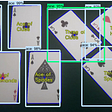## Grid searching Time Series Models## Assessing the impacts of the Privacy Sandbox piece by piece #1: Bring the noise## Principal Component Analysis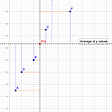## Twitter U.S. Airline Sentiment Analysis using Keras and RNNs## k-Means Clustering in BigQuery now does better initialization## The AutoTest Framework Makes the Operator Alignment Task for Deep Learning Frameworks Easy## Train BigBird Language model from scratch## OneFlow

OneFlow is a performance-centered and open-source deep learning framework.

## TorchMetrics v0.9 — Faster forward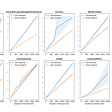## The Execution Process of a Tensor in a Deep Learning Framework## ViViT 📹 Video Vision Transformer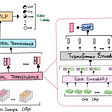## How to Reduce the Performance Gap Between GPU and CPU for Deep Learning Models